Hits: 0

# DFS Algorithm

#### In this tutorial, you will learn what a DFS algortihm is. Also, you will find working examples of DFS algorithm in C, C++, Java and Python.

Traversal means visiting all the nodes of a graph. Depth first traversal or Depth first Search is a recursive algorithm for searching all the vertices of a graph or tree data structure. In this article, you will learn with the help of examples the DFS algorithm, DFS pseudocode, and the code of the depth first search algorithm with implementation in C++, C, Java, and Python programs.

## DFS algorithm

A standard DFS implementation puts each vertex of the graph into one of two categories:

1. Visited
2. Not Visited

The purpose of the algorithm is to mark each vertex as visited while avoiding cycles.

The DFS algorithm works as follows:

1. Start by putting any one of the graph’s vertices on top of a stack.
2. Take the top item of the stack and add it to the visited list.
3. Create a list of that vertex’s adjacent nodes. Add the ones which aren’t in the visited list to the top of the stack.
4. Keep repeating steps 2 and 3 until the stack is empty.

## DFS example

Let’s see how the Depth First Search algorithm works with an example. We use an undirected graph with 5 vertices.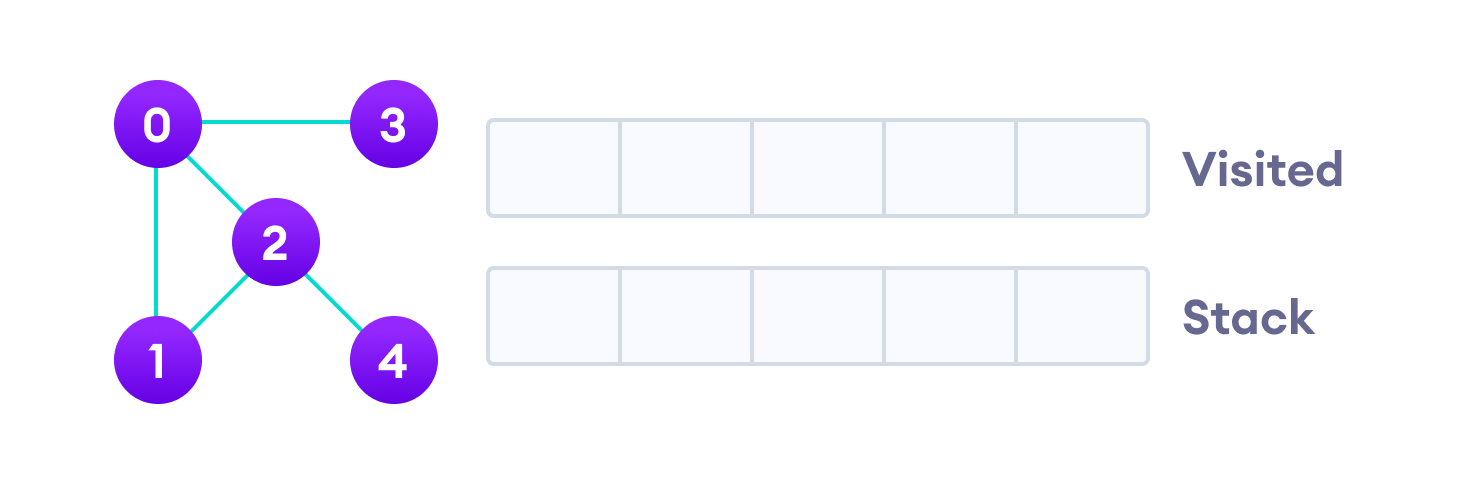Undirected graph with 5 vertices

We start from vertex 0, the DFS algorithm starts by putting it in the Visited list and putting all its adjacent vertices in the stack.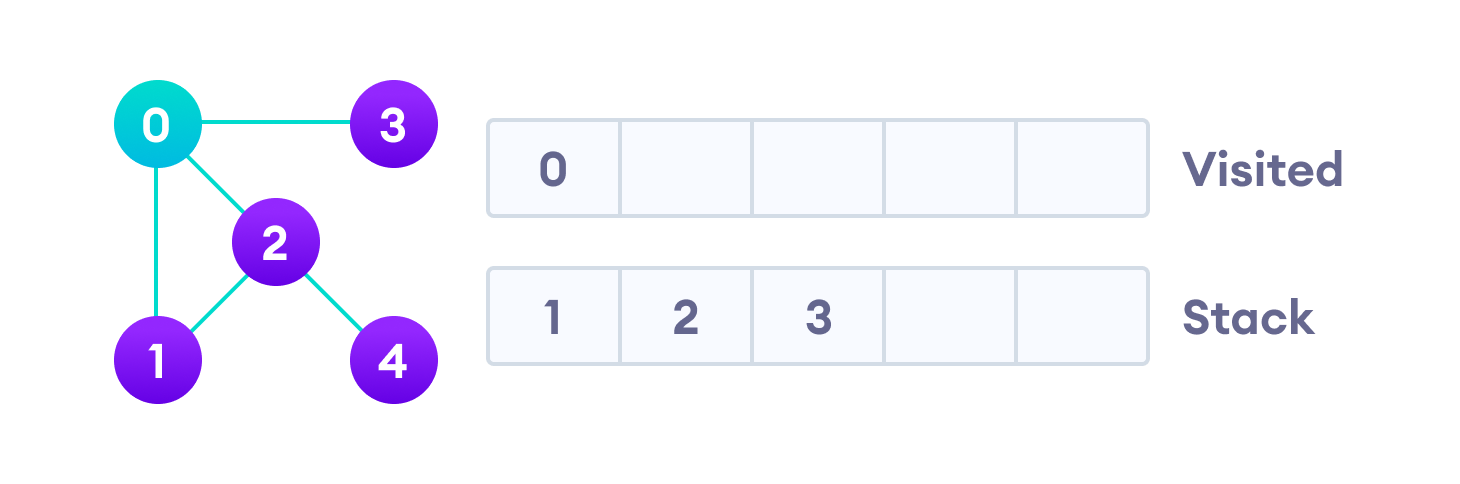Visit the element and put it in the visited list

Next, we visit the element at the top of stack i.e. 1 and go to its adjacent nodes. Since 0 has already been visited, we visit 2 instead.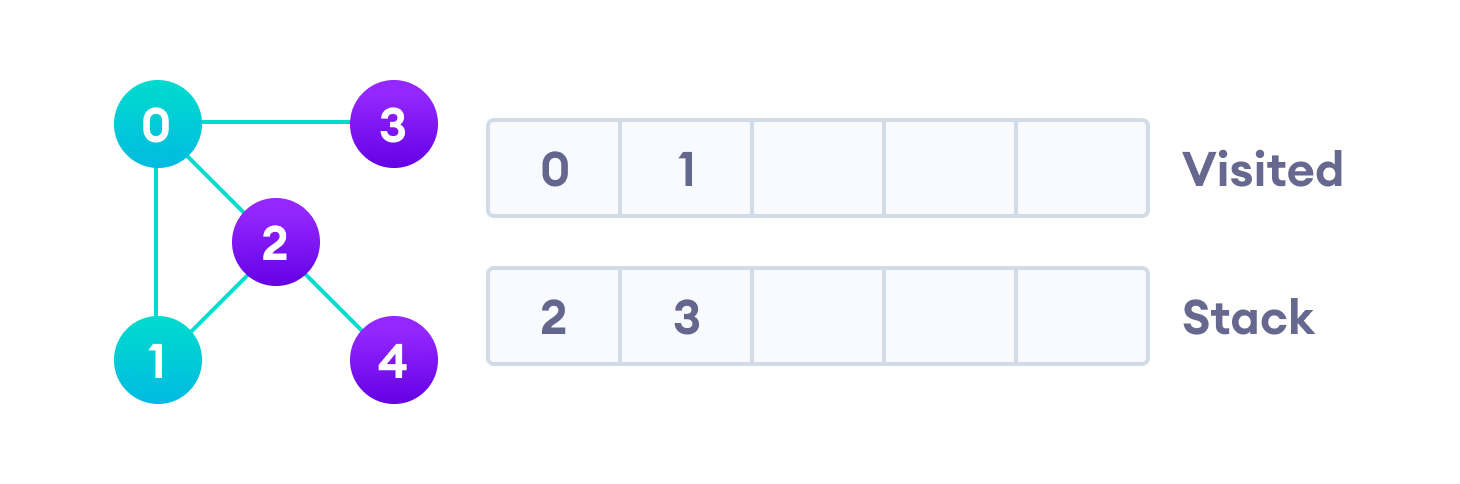Visit the element at the top of stack

Vertex 2 has an unvisited adjacent vertex in 4, so we add that to the top of the stack and visit it.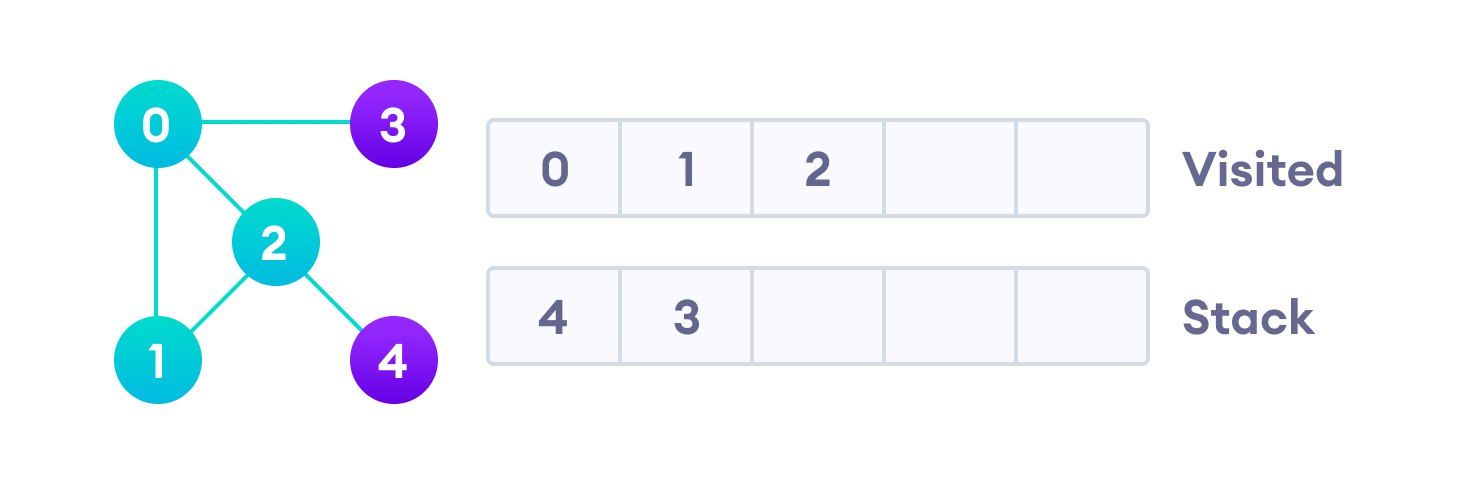Vertex 2 has an unvisited adjacent vertex in 4, so we add that to the top of the stack and visit it.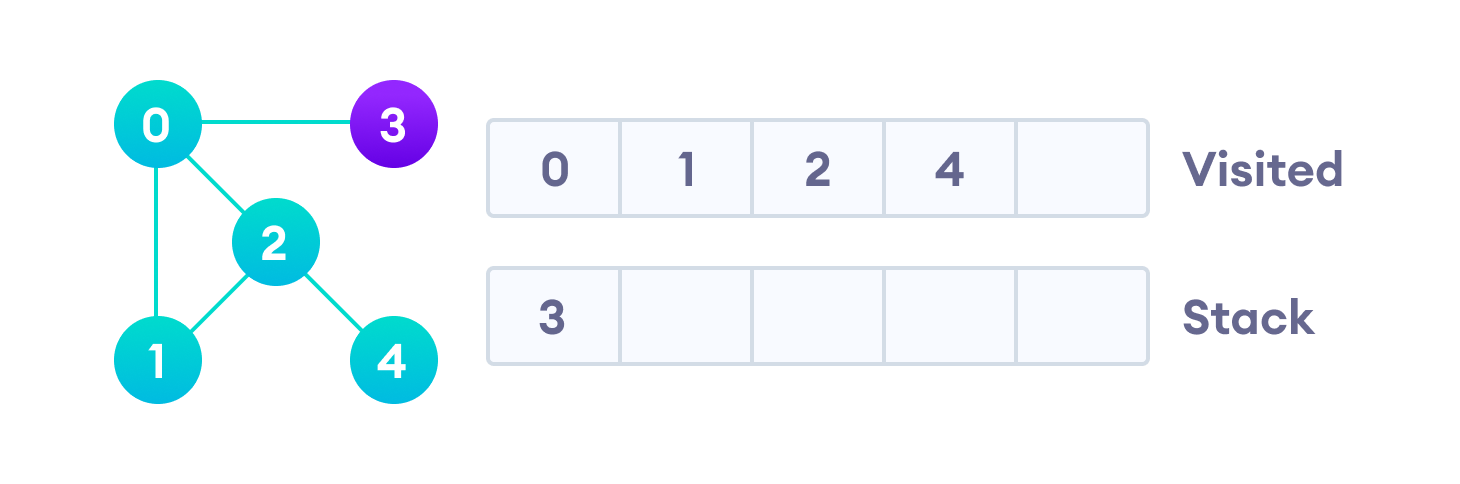Vertex 2 has an unvisited adjacent vertex in 4, so we add that to the top of the stack and visit it.

After we visit the last element 3, it doesn’t have any unvisited adjacent nodes, so we have completed the Depth First Traversal of the graph.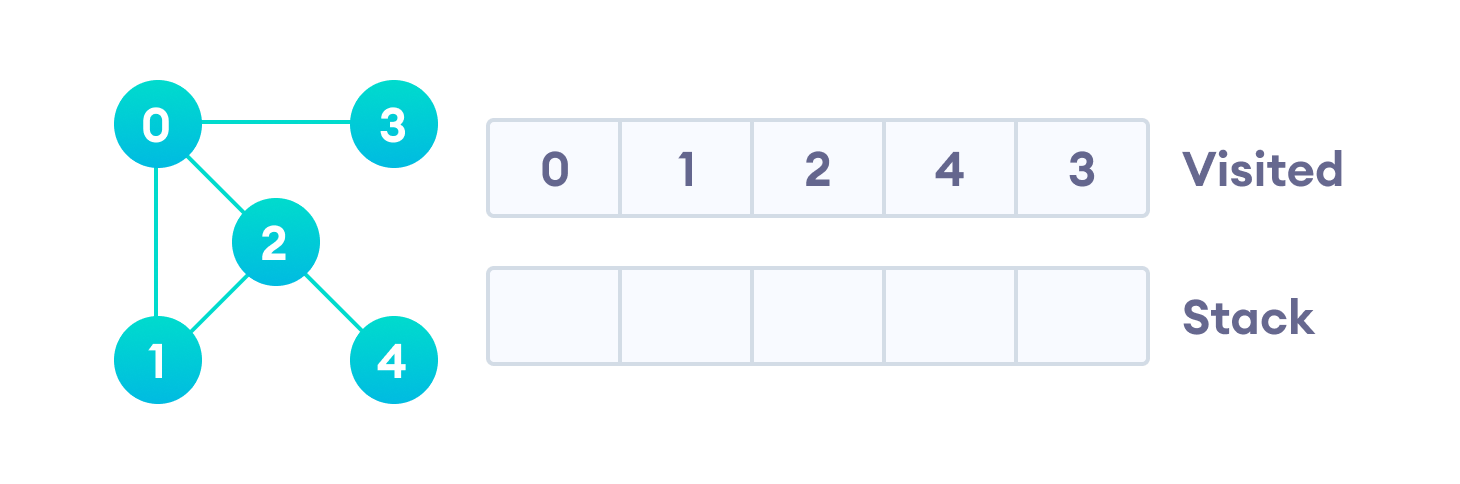After we visit the last element 3, it doesn’t have any unvisited adjacent nodes, so we have completed the Depth First Traversal of the graph.

## DFS pseudocode (recursive implementation)

The pseudocode for DFS is shown below. In the init() function, notice that we run the DFS function on every node. This is because the graph might have two different disconnected parts so to make sure that we cover every vertex, we can also run the DFS algorithm on every node.

```DFS(G, u)
u.visited = true
for each v ∈ G.Adj[u]
if v.visited == false
DFS(G,v)

init() {
For each u ∈ G
u.visited = false
For each u ∈ G
DFS(G, u)
}```

## Python Examples

The code for the Depth First Search Algorithm with an example is shown below. The code has been simplified so that we can focus on the algorithm rather than other details.

``````/* DFS algorithm in Python */

/* DFS algorithm */
def dfs(graph, start, visited=None):
if visited is None:
visited = set()
visited.add(start)

print(start)

for next in graph[start] - visited:
dfs(graph, next, visited)
return visited

graph = {'0': set(['1', '2']),
'1': set(['0', '3', '4']),
'2': set(['0']),
'3': set(['1']),
'4': set(['2', '3'])}

dfs(graph, '0')``````

## DFS Algorithm Complexity

The time complexity of the DFS algorithm is represented in the form of `O(V + E)`, where `V` is the number of nodes and `E` is the number of edges.

The space complexity of the algorithm is `O(V)`.

## DFS Algorithm Applications

1. For finding the path
2. To test if the graph is bipartite
3. For finding the strongly connected components of a graph
4. For detecting cycles in a graph

# Special 95% discount

## 2000+ Applied Machine Learning & Data Science Recipes

### Portfolio Projects for Aspiring Data Scientists: Tabular Text & Image Data Analytics as well as Time Series Forecasting in Python & R## Two Machine Learning Fields

There are two sides to machine learning:

• Practical Machine Learning:This is about querying databases, cleaning data, writing scripts to transform data and gluing algorithm and libraries together and writing custom code to squeeze reliable answers from data to satisfy difficult and ill defined questions. It’s the mess of reality.
• Theoretical Machine Learning: This is about math and abstraction and idealized scenarios and limits and beauty and informing what is possible. It is a whole lot neater and cleaner and removed from the mess of reality.

Data Science Resources: Data Science Recipes and Applied Machine Learning Recipes

Introduction to Applied Machine Learning & Data Science for Beginners, Business Analysts, Students, Researchers and Freelancers with Python & R Codes @ Western Australian Center for Applied Machine Learning & Data Science (WACAMLDS) !!!

Latest end-to-end Learn by Coding Recipes in Project-Based Learning:

Applied Statistics with R for Beginners and Business Professionals

Data Science and Machine Learning Projects in Python: Tabular Data Analytics

Data Science and Machine Learning Projects in R: Tabular Data Analytics

Python Machine Learning & Data Science Recipes: Learn by Coding

R Machine Learning & Data Science Recipes: Learn by Coding

Comparing Different Machine Learning Algorithms in Python for Classification (FREE)

`Disclaimer: The information and code presented within this recipe/tutorial is only for educational and coaching purposes for beginners and developers. Anyone can practice and apply the recipe/tutorial presented here, but the reader is taking full responsibility for his/her actions. The author (content curator) of this recipe (code / program) has made every effort to ensure the accuracy of the information was correct at time of publication. The author (content curator) does not assume and hereby disclaims any liability to any party for any loss, damage, or disruption caused by errors or omissions, whether such errors or omissions result from accident, negligence, or any other cause. The information presented here could also be found in public knowledge domains.  `